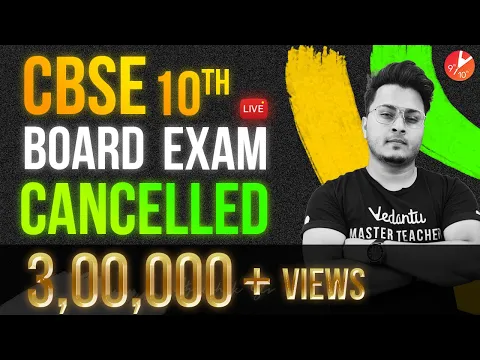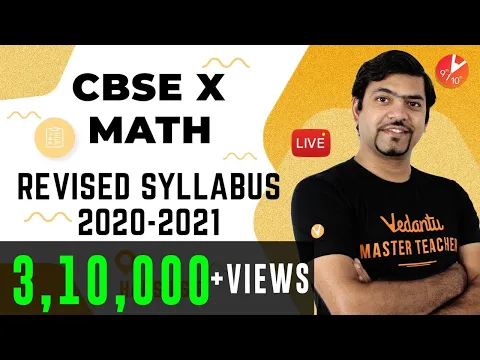Courses
Courses for Kids
Free study material
Offline Centres
More

# Maths Question Paper for CBSE Class 10 - 2011Last updated date: 03rd Dec 2023
Total views: 664.5k
Views today: 19.64k

## Previous Year Question Paper for CBSE Class 10 Maths - 2011 - Free PDF Download

Free download CBSE Class 10 Maths 2011 question paper with solutions solved by expert teachers. Register for Live Online Maths tuition to clear your doubts. Register Online for Class 10 Science tuition on Vedantu.com to score more marks in the CBSE board examination. Vedantu is a platform that provides free CBSE Solutions (NCERT) and other study materials for students. Maths Students who are looking for better solutions can download Class 10 Maths NCERT Solutions to help you to revise the complete syllabus and score more marks in your examinations.

Watch videos on
Maths Question Paper for CBSE Class 10 - 2011CBSE Class 10 Result 2022 Declared | CBSE Exam Results LIVE | Term 2 CBSE Results 2022| Vedantu 9&10
Vedantu 9&10
SubscribeShare
2.5K likes
88K Views
1 year agoClass 10 Maths Paper Presentation Tips 2022: ✔️DO's and ❌Don'ts by Harsh Sir | Vedantu 9 & 10
Vedantu 9&10
4.9K likes
61.4K Views
1 year ago
Take TestCBSE 10th Board Exam Cancelled 🔥 | CBSE Latest Update in Detail | NO Rumors |ONLY Facts|Abhishek Sir
Vedantu 9&10
30.3K likes
359.6K Views
2 years agoCBSE Maths Syllabus Reduction 2020 - 2021 | CBSE Class 10 Maths | Harsh Sir | Vedantu Class 9 & 10
Vedantu 9&10
9.4K likes
341K Views
3 years ago
See More## The need to solve the previous year’s question paper for CBSE Class 10 Maths

Solving the Class 10 previous year’s paper can prove to be very helpful, especially in a subject like Maths. This is because Maths requires practice to excel at. Solving previous year’s papers and sample papers boosts your confidence. If you ace at sample papers, you can find what chapters are your strengths in Class 10 Maths. If you solve the previous year’s paper and find out bad scores, you can again go through it and search for your mistakes to work on your weaknesses. With this practice eventually, you will avoid making the same mistake in your final board exam paper. Solving papers also aids in getting rid of exam-related fear.

### Paper pattern of CBSE Class 10 Maths

The Maths paper is divided into two main parts: PART A & PART B.

Further, PART A will have two sections:

1. Section I, this section will contain 16 questions. The student will get to choose between 5 internal choices in any of the questions. The 16 questions will be of 1 mark each.

2. Section II, this section will have 4 major questions, each question will be based on a case study. Each question will have 5 subparts. The student will have to answer any 4 subparts of the question from 5.  Each sub-question carries 1 mark.

PART B will have 16 questions in total.

• The First 6 questions will be very short answer types, each right answer will be worth 2 marks.

• 7 questions after this will carry 3 marks each and the student has to write at least 60 words in each answer.

• The last 3 questions will be long answer type, each will carry 5 marks each.

• In this PART internal choices will be given, 2 in the 2 mark questions, 2 in the 3 mark questions, and 1 in the 5 mark questions.

Download CBSE Class 10 2011 maths question paper and solution to practice and find out how the experts have answered the questions following the guidelines of CBSE. Learn how to approach these questions and focus on the format followed by the board. Keep practicing and refer to the study materials offered by Vedantu for Class 10 maths and develop a strong conceptual foundation.

## FAQs on Maths Question Paper for CBSE Class 10 - 2011

1. Where can I find resources for CBSE Class 10 Maths?

You can find various resources for CBSE Class 10 Maths on Vedantu. Vedantu is a platform that provides on-point notes, sample papers, video lectures, which are required to ace the CBSE Class 10 Maths exam. You can blindly rely on the content on Vedantu as it is precisely curated for students to refer to and learn from. The NCERT textbook is also a good resource to study from, as it covers all the topics of the syllabus.

2. How to score well in the CBSE Class 10 Maths paper?

Maths is all about practice. The more you practice, the more you understand the subject well and as a result, the better you score. To score good marks in board exams you must prepare well. Try making notes for every chapter. Make a list of formulas for every chapter in Maths and have a look at them every day. Solve your NCERT exercises, there is a possibility most of the questions in your exams will be related to them. Try revising and re-revising once you have prepared. Solve as many practice and sample papers and previous year question papers as possible.

3. What are composite numbers as asked in the previous year’s question paper for CBSE Class 10 Maths?

Composite numbers are numbers that we get when we multiply two numbers smallest positive integers, they contain at least one divisor other than number 1. The two numbers that are used to obtain a composite number are known as factors. So, it is said that composite numbers are numbers that have two factors. For instance, 6 is a composite number and its two factors are 2 and 3. On the other hand, 0 cannot be called a composite number as it does have any factors.

4. According to CBSE Class 10 Maths book, what are prime numbers?

Prime numbers are numbers that we cannot get when we multiply any two numbers other than the number itself. Prime numbers are also known as numbers that aren’t divisible by any other number than number 1 and themselves. Prime numbers are also defined as positive integers that cannot be a product of any other two positive integers. For instance, 7 is a prime number as there are no two positive integers that can be multiplied to get 7 as a product. Whereas 6 is not a prime number as 2 and 3 can be multiplied to obtain 6 as a product.

5. What are real numbers according to the CBSE Class 10 Maths book?

Real numbers are those that one can represent on a number line. They are the most used numbers in Maths. Rational numbers and Irrational numbers together are known as real numbers. There are various types of real numbers. Natural numbers, Integers, Whole numbers, rational numbers, and irrational numbers are all types of real numbers. Real numbers have commutative properties for both multiplication and addition, this means that while multiplying and summing up two numbers there place doesn’t make a difference, the answer is going to remain the same. For instance, 23 is 6, and 32 is also 6.